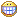# Create Simple Login Form Using PHP [Basic]

Hello...
How are you.....The post before, the writter has sharing How to Create Simple Comment Form Using PHP. That posting has been implemented the lesson of input output using PHP MySQL. For the next lesson, the writter will be share how to Create Simple Login Form Using PHP. This lesson is basic of login method in website.

Okay, prepare in this lesson is, Web Server and MySQL, you can use XAMPP on this condition.

Let's go to the Lesson,

First, you could create a Database on MySQL and one table. There are 3 field on the table. Follow this tutorial for simple Lesson.
Now, the writter create a Database with name is blog. Than create table with the name is user. Next, there are 3 fields on the user table, it is a id_user as Primary Key,  username char(32), and password char(32). For detail, look at below.

That's a structure of Fields on the user table. After you create a Database, Table, And Fields. Now you must fiil the Fields. For expample look at below.

Okay, now we just need a 2 (Two) Pages, 1 (one) for Login Page, dan 1 (one) again for Checking Page.

Let's create a Login Page, this page we will give the name is login.html. Below is the simple script on login page.

<html>
<head>
<title>Login Form</title>
</head>
<body>
<h2>Login Form</h2>
<br>
<form method="POST" action="check.php">
<table>
<tr>
<td>Username</td>
<td><input type="text" name="user" size="20"></td>
</tr>
<tr>
<td>Password</td>
<td><input type="password" name="pass" size="20"</td>
</tr>
<tr>
<td>&nbsp;</td>
<td><input type="submit" name="submit" value="Login">&nbsp;<input type="reset" name="reset" value="Reset"></td>
</tr>
</table>
</form>
</body>
And than, create the checking page with the name is check.php. Below is the simple script on checking page.

<?php

##### Connection to database #####
\$hostname = "localhost";
\$username = "root";
\$password = "";
\$dbname = "blog";
\$link = mysql_connect(\$hostname,\$username,\$password) or die("Error Connection");
mysql_select_db(\$dbname,\$link);
##### End #####

##### Receive Input #####
\$user = \$_POST['user'];
\$pass = \$_POST['pass'];
##### End #####

##### Checking input in table within database #####
\$sql = "select * from user where username = '\$user' AND password = '\$pass'";
\$hasil = mysql_query(\$sql) or die("wrong");
\$row = mysql_fetch_array(\$hasil);
if(\$row == "0") {
echo "<h2>Sorry, username and password does not match</h2>";
}
elseif(\$row != "0"){
echo "<h2>Login is Successful....</h2>";
}
##### End #####

?>
If all scripts was created. The simple login form was already to use.
The explain of that script is below.

\$hostname = "localhost";
\$username = "root";
\$password = "";
\$dbname = "blog";
\$link = mysql_connect(\$hostname,\$username,\$password) or die("Error Connection");
mysql_select_db(\$dbname,\$link);

it's script, are use to create connection between page to database.

\$user = \$_POST['user'];
\$pass = \$_POST['pass'];
it's use to receive a input from html page (input username and password). After receive, the value will be include in the variables (\$user and \$pass).

\$sql = "select * from user where username = '\$user' AND password = '\$pass'";
\$hasil = mysql_query(\$sql) or die("wrong");
\$row = mysql_fetch_array(\$hasil);
it's use to checking value of \$user and \$pass on the Database. The basic of this process is on the query syntax. And than the query is executed with mysql_query(); syntax. And than will processed with mysql_fetch_array(); syntax. The result of it will be included on the \$row variable. The value of the \$row variable is one or zero.

if(\$row == "0") {
echo "<h2>Sorry, username and password does not match</h2>";
}
elseif(\$row != "0"){
echo "<h2>Login is Successful....</h2>";
}

It's to use to checking a value of the \$row variable, is the value zero or one. If zero means username and password does not match. And if one means username and password does match.

Let's try it.
First. open the login.html page.

Than, fill username and password, than click "Login"
If, success you will look like below

if username and password does not match, you will look at below

Okay, that is Basic of Login Form Using PHP.
On the next time, the writter will share for Advanced Login Form Using PHP.

Happy Paper 4share !!